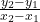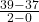## A ball thrown in the air has a height of y = – 16x² + 50x + 3 feet after x seconds. a) What are the units of measurement for the rate of cha

Question

A ball thrown in the air has a height of y = – 16x² + 50x + 3 feet after x seconds. a) What are the units of measurement for the rate of change of y? b) Find the rate of change of y between x = 0 and x = 2?

in progress 0
2 weeks 2021-09-02T19:55:59+00:00 1 Answers 0 views 0

(a) ft/s

(b) 1ft/s

## Step-by-step explanation:

Given equation;

y = (- 16x² + 50x + 3)ft       ————-(i)

Where;

y is measured in feet(ft)

x is measured in seconds(s).

(a) The rate of change of y with respect to x is found by dividing the total change in y by the total change in x. i.e

Δy / Δx

Where;

Δy = y₂ – y₁

Δx = x₂ – x₁

∴ Δy / Δx =————–(ii)

Since y is measured in feet, Δy will also be measured in feet.

Also, since x is measured in seconds, Δx will also be measured in seconds.

Therefore, the rate of change of y with respect to x (Δy / Δx) will be measured in feet per second (ft/s)

(b) The rate of change of y between x  = 0 and x = 2 can be found by using equation (ii)

Where;

y₂ is the value of y at x = 2 found by substituting x = 2 into equation (i)

y₁ is the value of y at x = 0 found by substituting x = 0 into equation (i)

=> y₂ =  – 16(2)² + 50(2) + 3 = 39

=> y₁ =  – 16(1)² + 50(1) + 3 = 37

Now, substitute the values of y₂, y₁, x₂ and x₁  into equation (ii)

Δy / Δx =Δy / Δx =Δy / Δx = 1

Therefore, the rate of change of y is 1 ft/s